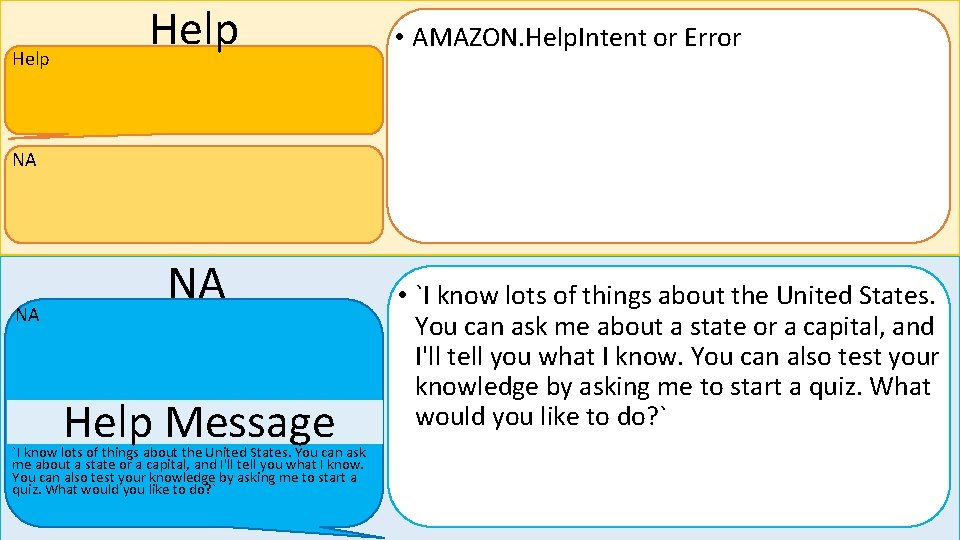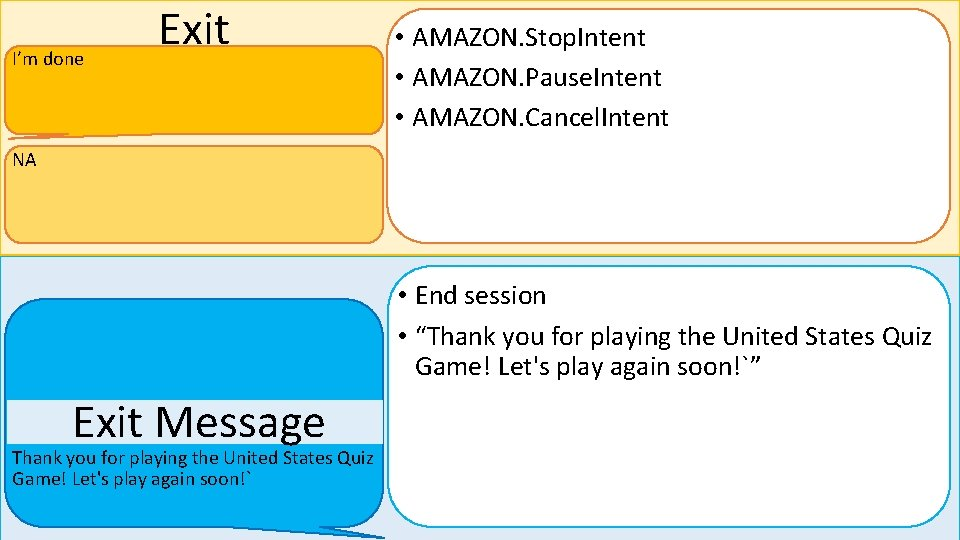# Quiz Skill Overview This skill teaches and quizzes

• Slides: 13Quiz Skill Overview This skill teaches and quizzes customers about the 50 States. It teaches about the state name, capital, abbreviation, year founded, and statehood order. Users can take a 10 question quiz to test their knowledge or ask Alexa about the points listed above to learn about a state. • • • Welcome Teach / define Quiz Error response (no slots matched) Help What’s my score?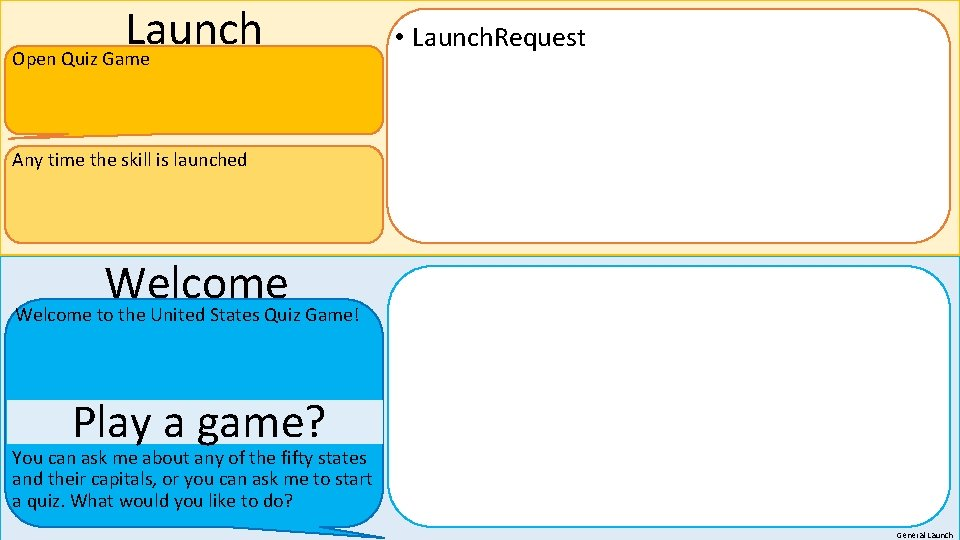Launch Open Quiz Game • Launch. Request Any time the skill is launched Welcome to the United States Quiz Game! Play a game? You can ask me about any of the fifty states and their capitals, or you can ask me to start a quiz. What would you like to do? General Launch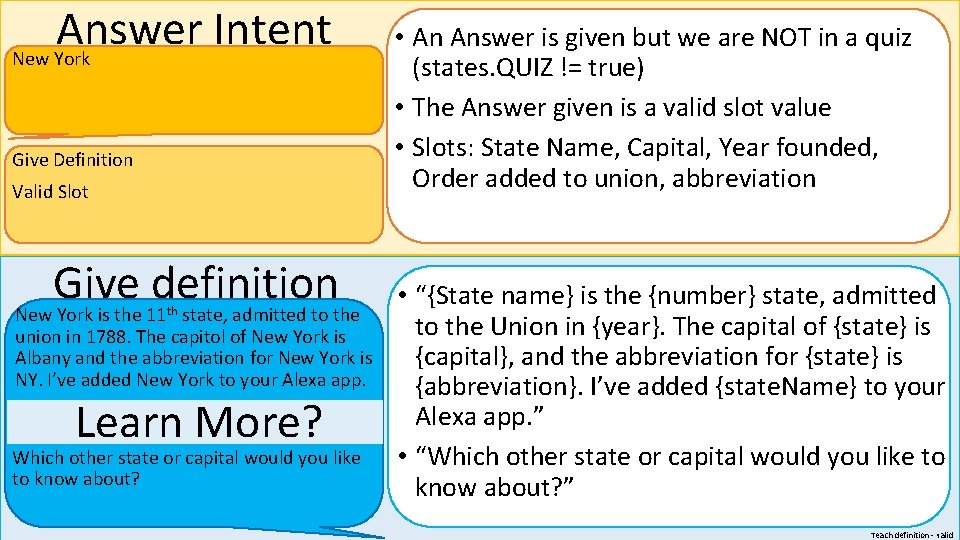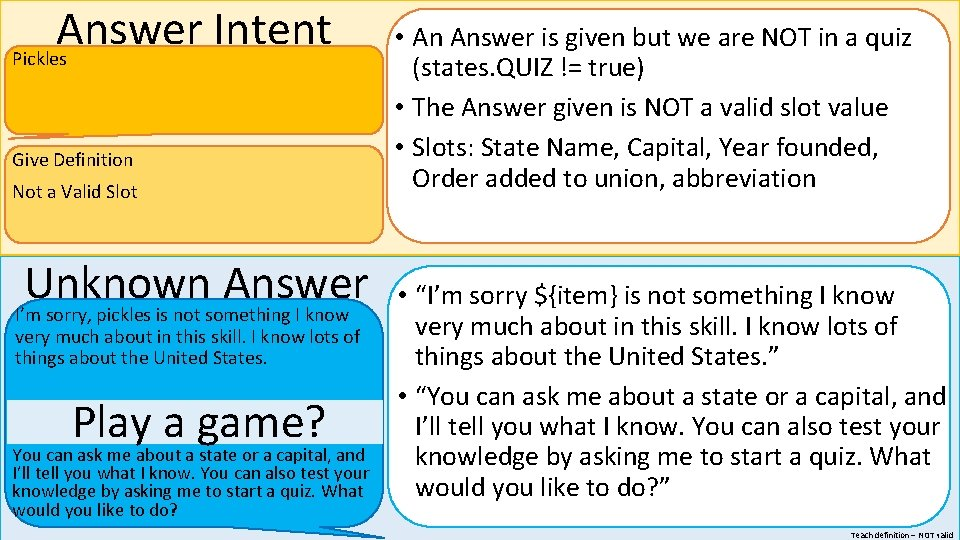Repeat as many times as the user would like, until they end the session or start a quiz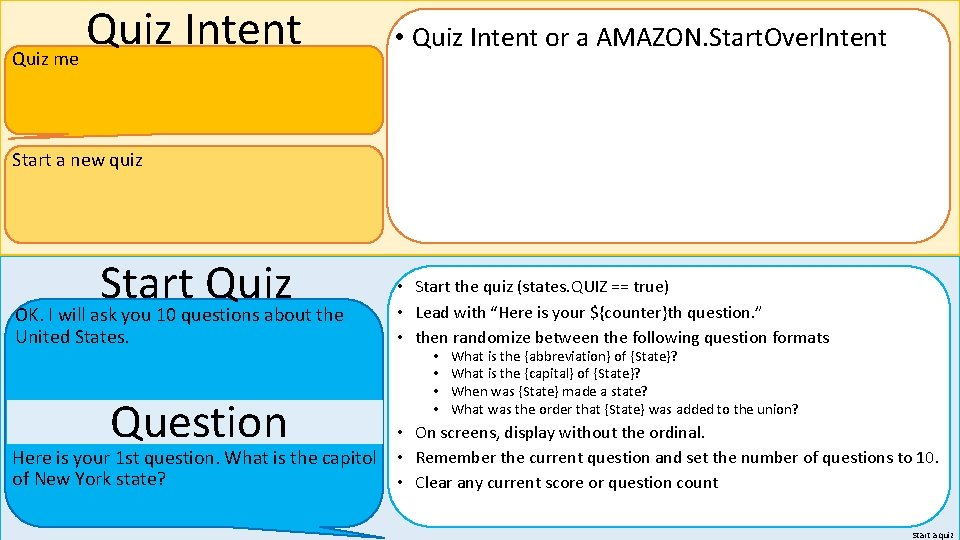Quiz Intent Quiz me • Quiz Intent or a AMAZON. Start. Over. Intent Start a new quiz Start Quiz OK. I will ask you 10 questions about the United States. Question Here is your 1 st question. What is the capitol of New York state? • Start the quiz (states. QUIZ == true) • Lead with “Here is your \${counter}th question. ” • then randomize between the following question formats • • What is the {abbreviation} of {State}? What is the {capital} of {State}? When was {State} made a state? What was the order that {State} was added to the union? • On screens, display without the ordinal. • Remember the current question and set the number of questions to 10. • Clear any current score or question count Start a quiz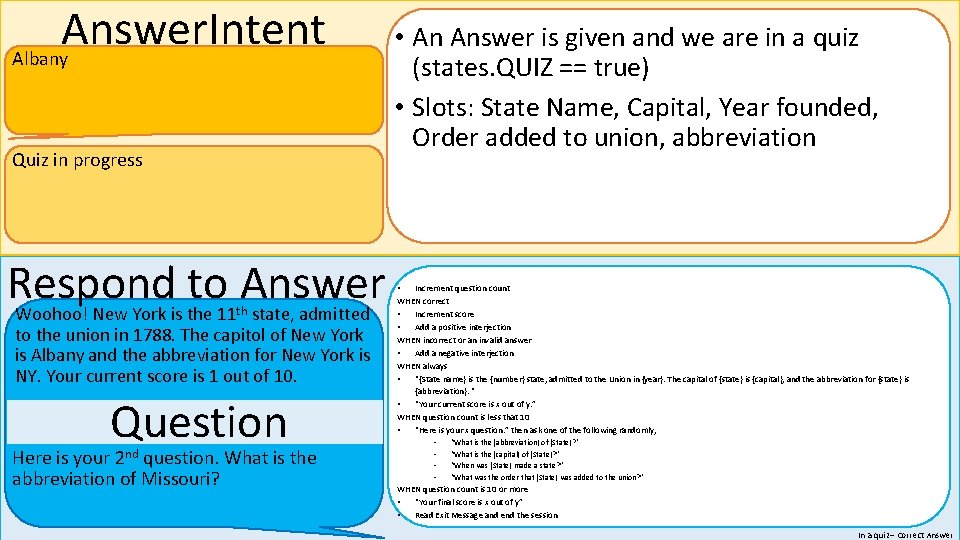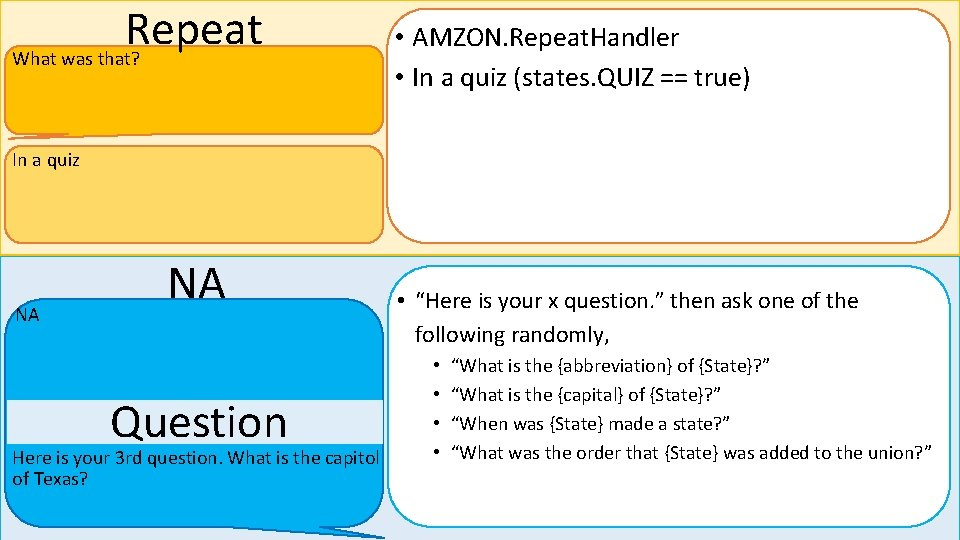Repeat What was that? • AMZON. Repeat. Handler • In a quiz (states. QUIZ == true) In a quiz NA NA Question Here is your 3 rd question. What is the capitol of Texas? • “Here is your x question. ” then ask one of the following randomly, • • “What is the {abbreviation} of {State}? ” “What is the {capital} of {State}? ” “When was {State} made a state? ” “What was the order that {State} was added to the union? ”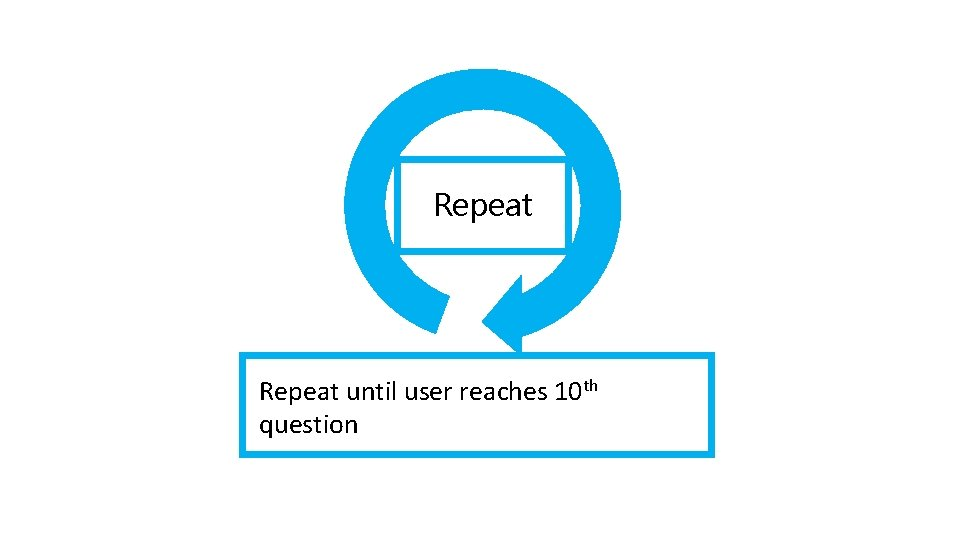repeat Repeat until user reaches 10 th question